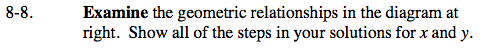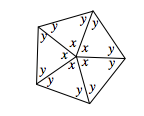Home > GC > Chapter 8 > Lesson 8.1.1 > Problem8-8

8-8.

the geometric relationships in the diagram at right. Show all of the steps in your solutions for x and y. Homework Help ✎x + x + x + x + x = 360° , because all of the central angles of the figure total 360°.

2y + x = 180°, because the Triangle Sum Theorem states the angles of a triangle total 180°.

x = 72°, y = 54°